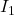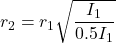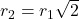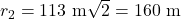## After your school’s team wins the regional championship, students go to the dorm roof and start setting off fireworks rockets. The rockets e

Question

After your school’s team wins the regional championship, students go to the dorm roof and start setting off fireworks rockets. The rockets explode high in the air and the sound travels out uniformly in all directions. If the sound intensity is 1.97 10-6 W/m2 at a distance of 113 m from the explosion, at what distance from the explosion is the sound intensity half this value?

in progress 0
2 weeks 2021-09-02T06:18:39+00:00 2 Answers 0 views 0

Distance ,d= 159.81m

Explanation:

The intensity, I, of the sound is inversely proportional to the square of the distance, d,from the source.

Using the equation d/do=sqrt2

d= dosqrt2

Where d=113m

d= 113sqrt2

d= 159.81m

160 m

Explanation:

The intensity, I, of the sound is inversely proportional to the square of the distance, r, from the source.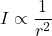Hence,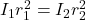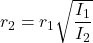From the question,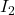is half of